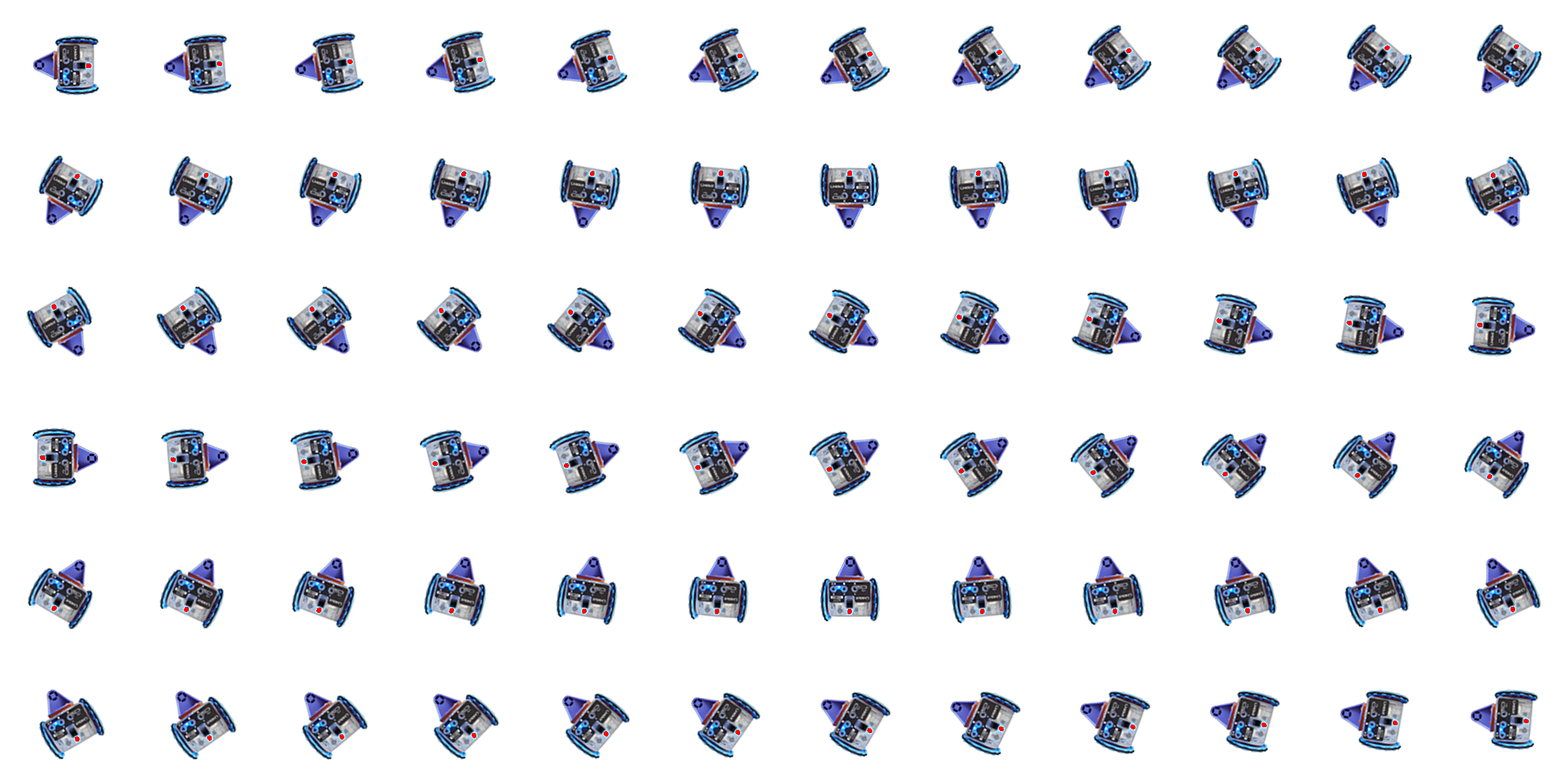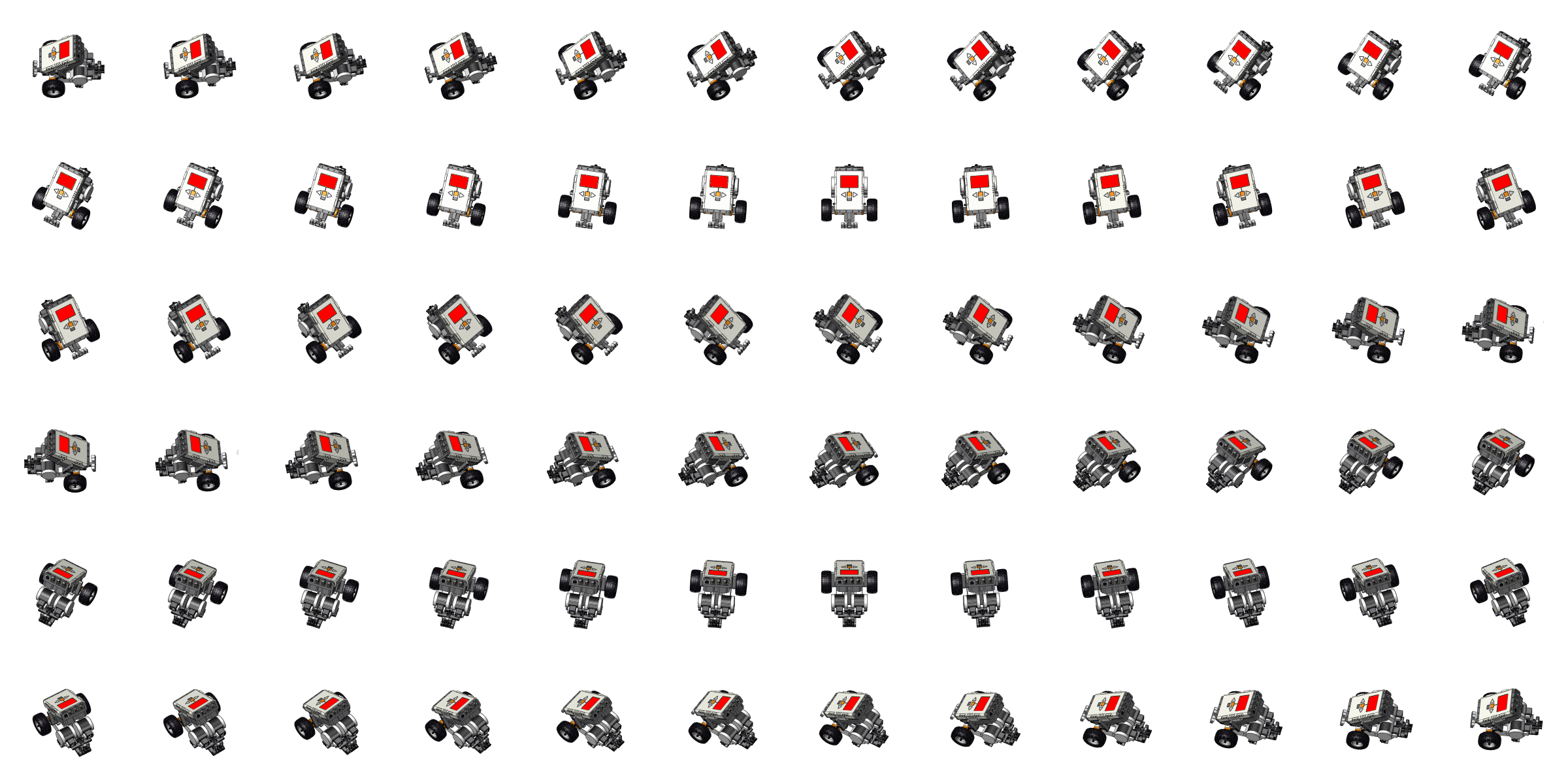Learning Math with Coding and RoboticsGrid Size: S M L Simple View: MinView:
Snowball Rolling Down a Mountain
 The pre-placed block draws the snowball at the point (1,17) on the top of the mountain with a radius of 0.25. Adjust the values so that the snowball will move in a diagonal direction down the mountain. The $$x$$ value should be set to equal the variable $$i$$ . The $$y$$ value should start at 17 and decrease by $$i$$ each time the loop iterates. The snowball radius should start at 0.25 and increase by $$i*0.5$$ each time the loop iterates.
 Piano Symbol Word APCSP Hardware LArduino Workspace Load Save Ch Ch Shell Console
Lock
roboblockyA180.chTime